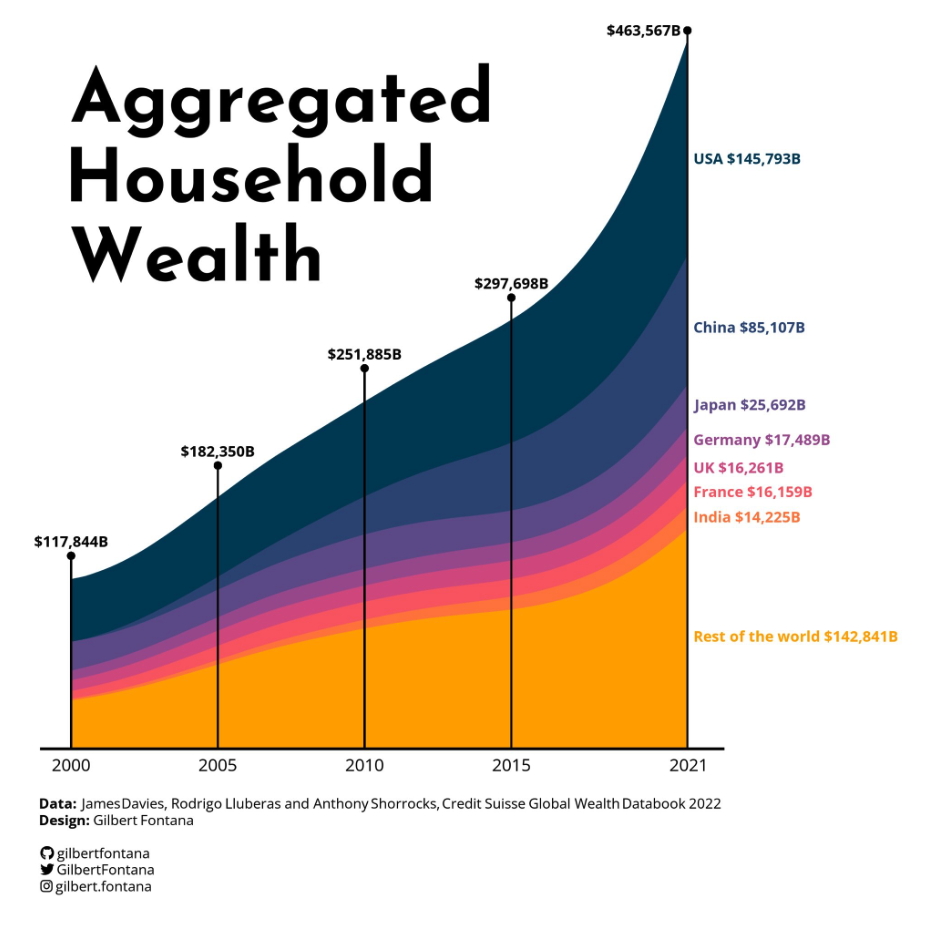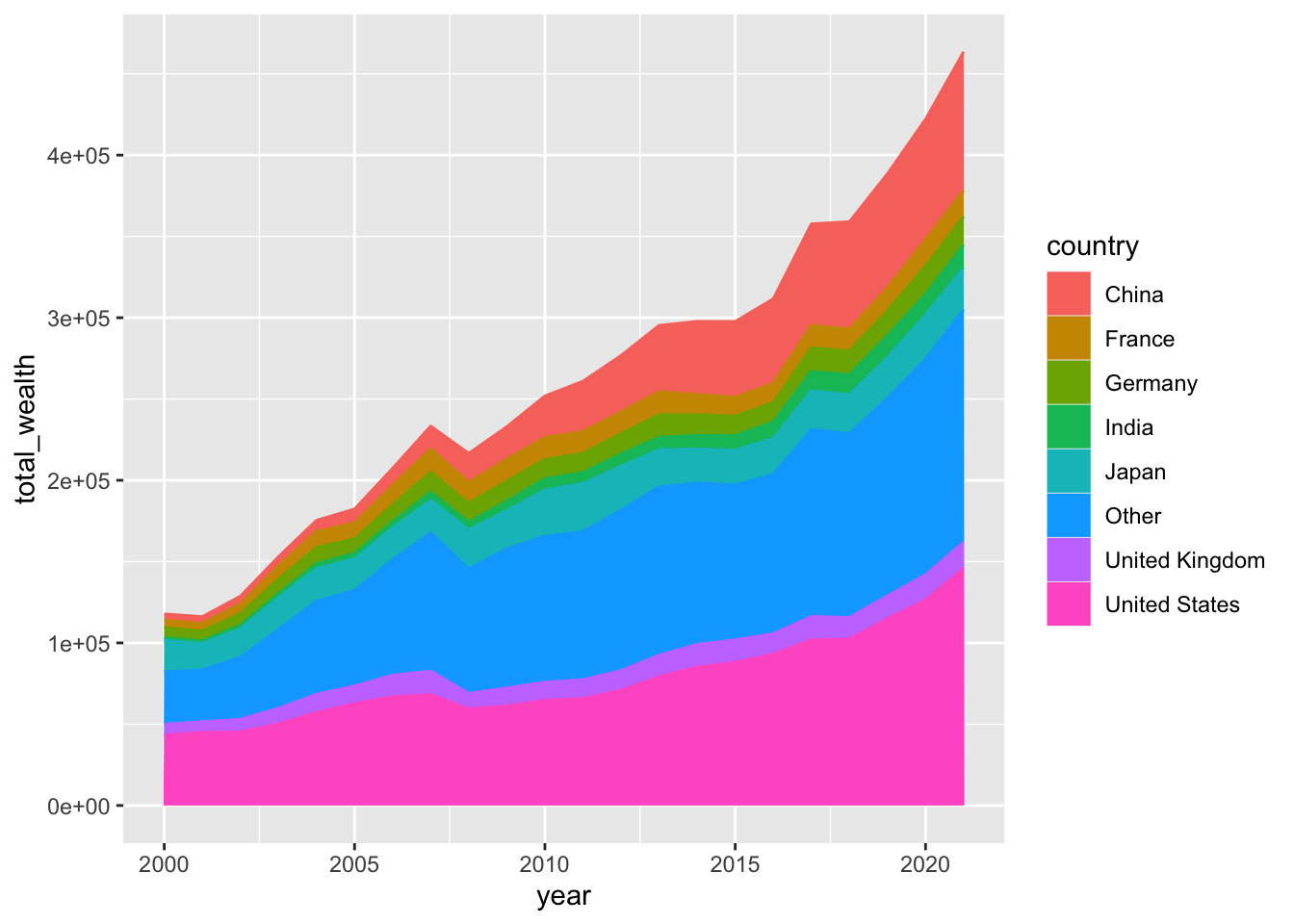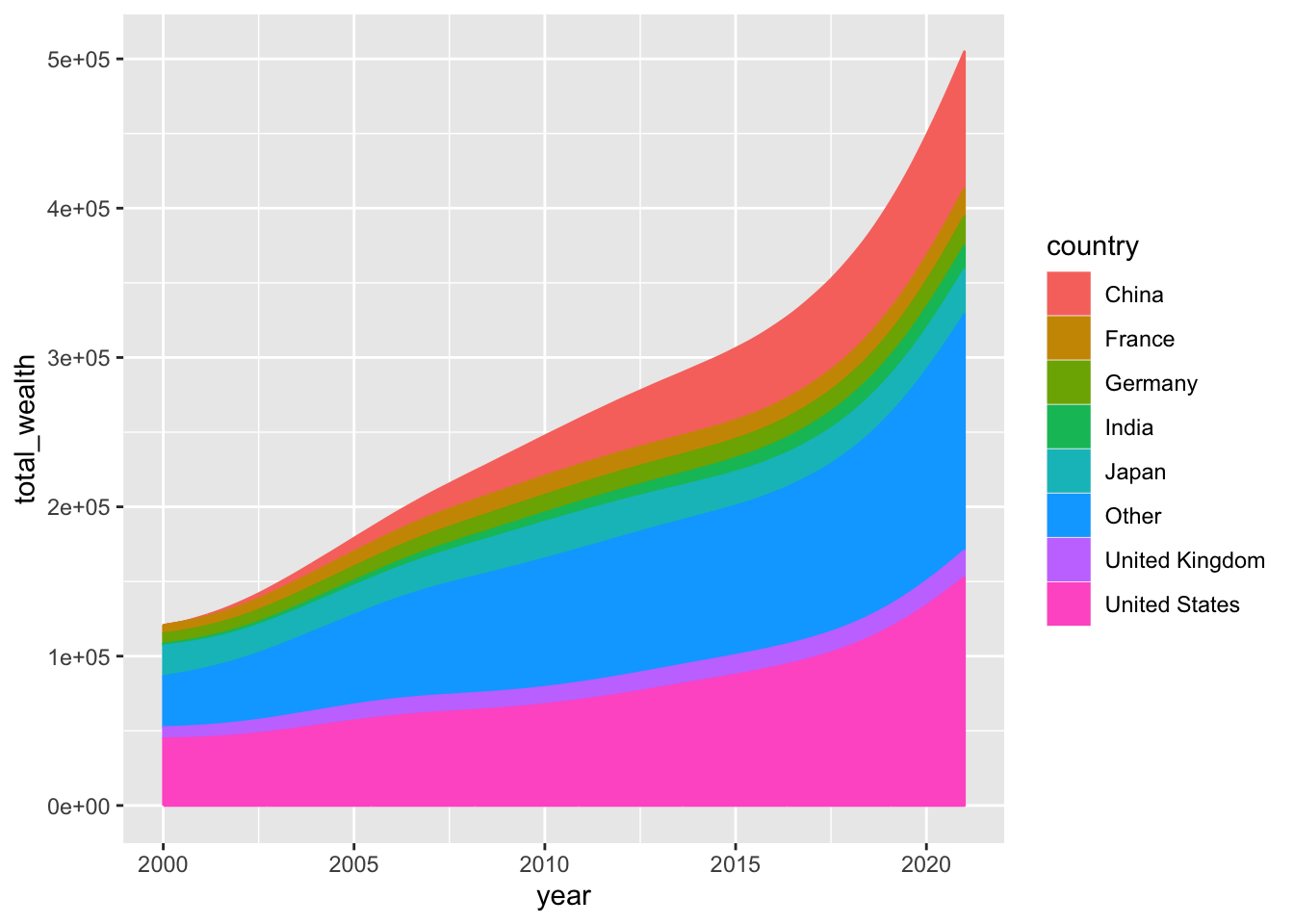# Stacked line chart with inline labels

This post shows how to build a clean stacked area chart using `R`, `ggplot2` and the `tidyverse`. This chart is ready for publication. It has a nice color palette, some very clean inline labels, a powerfull title and slick footer caption with logos.

Thanks to him for accepting sharing his work here! As a teaser, here is the plot we’re gonna try building:# Required packaged

Today’s plot requires quite a lot of packages to be built. You can install them with the `install.packages()` function. Once installed, load them with the `library()` function:

``````library(tidyverse)
#library(janitor)
library(ggstream)
library(showtext)
library(ggtext)``````

You are probably familiar with the `tidyverse` already. `readxl` will be used in the next section to load the dataset from a `xlsx` format directly. `ggstream` is used to smooth the area shapes. `showtext` is used to load some custom fonts.

# Load and prepare the data

The data is stored on github in a `xslx` file. To reproduce this tutorial, please download the file and run the following line of code. The `read_excel()` function of the `readxl` package makes it easy to load this file directly without requiring the `.csv` format.

``df <- read_excel("~/Desktop/R-graph-gallery/DATA/wealth_data.xlsx")``

Note: do not forget to update the path to point to the file on your computer.

# Basic stacked area chart

Everything start with a basic stacked area chart. You can see many examples in the stacked area chart section of the R graph gallery, including beginner level tutorials.

Basically, the `ggplot` function is used to start a chart with `ggplot2`. Then, the `year` column of the dataset (`df`) is used for the `x` axis, `total_wealth` for the Y axis and everything is stacked and colored using the `country` column.

Last but not least, the `geom_area` geom can be used to create a stacked area chart.

``````# Stacked area chart with smoothing
df %>%
ggplot(aes(year, total_wealth, fill = country, label = country, color = country)) +
geom_area() ``````That’s it! 🔥 We now have a first stacked area chart showing what’s happening in our dataset.

# Smoothing the lines

It is possible to make the lines smoother thanks to the `geom_stream` geom of the `ggstream` package. It’s gonna create a less accurate but more organic and eye catching shape to the graph:

``````# Stacked area chart with smoothing
df %>%
ggplot(aes(year, total_wealth, fill = country, label = country, color = country)) +
geom_stream(type = "ridge", bw=1) ``````# Color scale and stacking order

What looks especially good in Gilbert’s chart is the color palette. Let’s build a vector of color that we then inject into the chart using the `scale_fill_manual` and `scale_color_manual` functions:

``````#Color palette
pal=c("#003f5c",
"#2f4b7c",
"#665191",
"#a05195",
"#d45087",
"#f95d6a",
"#ff7c43",
"#ffa600")

# Stacking order
order <- c("United States", "China", "Japan", "Germany", "United Kingdom", "France", "India", "Other")

# Use them for the plot
df %>%
arrange(total_wealth) %>%
mutate(country = factor(country, levels=order)) %>%
ggplot(aes(year, total_wealth, fill = country, label = country, color = country)) +
geom_stream(type = "ridge" ,bw=1) +

scale_fill_manual(values=pal) +
scale_color_manual(values=pal) +
scale_x_continuous(breaks=c(2000,2005,2010,2015,2021),labels = c("2000","2005","2010","2015","2021")) +
scale_y_continuous(expand = c(0,0)) +
coord_cartesian(clip = "off") ``````# Using custom fonts

Before adding the title, legend and inline labels we need to load some custom fonts.

This is made possible thanks to the `showtext` package and its `font_add_google()` function. Using custom fonts can be a bit tricky. Fortunately, I wrote a complete tutorial just in case the following code sounds strange to you.

``````# Name of the fonts we need
font <- "Josefin Sans"
font2 <- "Open Sans"

fa_path <- systemfonts::font_info(family = "Font Awesome 6 Brands")[["path"]]
font_add(family = "fa-brands", regular = fa_path)

theme_set(theme_minimal(base_family = font2, base_size = 3))

bg <- "white"
txt_col <- "black"

showtext_auto(enable = TRUE)``````

Since we are talking about thext, let’s also create the caption text that appear at the bottom of the figure:

``````caption_text  <- str_glue("**Data:**  James Davies, Rodrigo Lluberas and Anthony Shorrocks, Credit Suisse Global Wealth Databook 2022<br>",
"**Design:** Gilbert Fontana <br><br>",
"<span style='font-family: \"fa-brands\"'>&#xf09b;</span> gilbertfontana<br>",
"<span style='font-family: \"fa-brands\"'>&#xf099;</span> GilbertFontana<br>",
"<span style='font-family: \"fa-brands\"'>&#xf16d;</span> gilbert.fontana"
)``````

# Color, legends, titles and labels

The final figure can now be created:

• the basic stacked area chart with great color is made as described above
• the title is used thanks to the `annotate()` function
• all inline labels are built 1 by 1, thanks to the `annotate()` function again
• vertical segments are made made one by one using the `geom_segment()` function
``````plot <- df %>%
arrange(total_wealth) %>%
mutate(country = factor(country, levels=order)) %>%
ggplot(aes(year, total_wealth, fill = country, label = country, color = country)) +
geom_stream(type = "ridge" ,bw=1) +

#Title
annotate("text", x = 2000, y = 410000,
label = "Aggregated\nHousehold\nWealth",
hjust=0,
size=15,
lineheight=.9,
fontface="bold", family=font,
color="black") +

#USA
annotate("text", x = 2021.2, y = 420000,
label = "USA \$145,793B",
hjust=0,
size=3,
lineheight=.8,
fontface="bold", family=font2,
color=pal) +
#China
annotate("text", x = 2021.2, y = 300000,
label = "China \$85,107B",
hjust=0,
size=3,
lineheight=.8,
fontface="bold",family=font2,
color=pal) +
#Japan
annotate("text", x = 2021.2, y = 245000,
label = "Japan \$25,692B",
hjust=0,
size=3,
lineheight=.8,
fontface="bold",family=font2,
color=pal) +
#Germany
annotate("text", x = 2021.2, y = 220000,
label = "Germany \$17,489B",
hjust=0,
size=3,
lineheight=.8,
fontface="bold",family=font2,
color=pal) +
#UK
annotate("text", x = 2021.2, y = 200000,
label = "UK \$16,261B",
hjust=0,
size=3,
lineheight=.8,
fontface="bold",family=font2,
color=pal) +
#France
annotate("text", x = 2021.2, y = 183000,
label = "France \$16,159B",
hjust=0,
size=3,
lineheight=.8,
fontface="bold",family=font2,
color=pal) +
#India
annotate("text", x = 2021.2, y = 165000,
label = "India \$14,225B",
hjust=0,
size=3,
lineheight=.8,
fontface="bold",family=font2,
color=pal) +
#Other
annotate("text", x = 2021.2, y = 80000,
label = "Rest of the world \$142,841B",
hjust=0,
size=3,
lineheight=.8,
fontface="bold",family=font2,
color=pal) +

## Vertical segments
geom_segment(aes(x = 2000, y = 0, xend = 2000, yend = 117426+20000),color="black") +
geom_point(aes(x = 2000, y = 117426+20000),color="black") +
annotate("text", x = 2000, y = 117426+30000,
label = "\$117,844B",
hjust=0.5,
size=3,
lineheight=.8,
fontface="bold",family=font2,
color=txt_col) +

geom_segment(aes(x = 2005, y = 0, xend = 2005, yend = 181731+20000),color="black") +
geom_point(aes(x = 2005, y = 181731+20000),color="black") +
annotate("text", x = 2005, y = 181731+30000,
label = "\$182,350B",
hjust=0.5,
size=3,
lineheight=.8,
fontface="bold",family=font2,
color=txt_col) +

geom_segment(aes(x = 2010, y = 0, xend = 2010, yend = 250932+20000),color="black") +
geom_point(aes(x = 2010, y = 250932+20000),color="black") +
annotate("text", x = 2010, y = 250932+30000,
label = "\$251,885B",
hjust=0.5,
size=3,
lineheight=.8,
fontface="bold",family=font2,
color=txt_col) +

geom_segment(aes(x = 2015, y = 0, xend = 2015, yend = 296203+25000),color="black") +
geom_point(aes(x = 2015, y = 296203+25000),color="black") +
annotate("text", x = 2015, y = 296203+35000,
label = "\$297,698B",
hjust=0.5,
size=3,
lineheight=.8,
fontface="bold",family=font2,
color=txt_col) +

geom_segment(aes(x = 2021, y = 0, xend = 2021, yend = 461370+50000),color="black") +
geom_point(aes(x = 2021, y = 461370+50000),color="black") +
annotate("text", x = 2021, y = 461370+50000,
label = "\$463,567B",
hjust=1.1,
size=3,
lineheight=.8,
fontface="bold",family=font2,
color=txt_col) +

#Color scale
scale_fill_manual(values=pal) +
scale_color_manual(values=pal) +
scale_x_continuous(breaks=c(2000,2005,2010,2015,2021),labels = c("2000","2005","2010","2015","2021")) +
scale_y_continuous(expand = c(0,0)) +

#Last customization
coord_cartesian(clip = "off") +
xlab("") +
ylab("") +
labs(caption = caption_text #"Data: Flash Eurobarometer, Number 509 (October 2022)"
) +
theme(
axis.line.x = element_line(linewidth = .75),
panel.grid = element_blank(),
axis.text.y=element_blank(),
axis.text.x = element_text(color=txt_col, size=10,margin = margin(5,0,0,0)),
plot.margin = margin(20,120,20,20),
legend.position = "none",
plot.caption = element_markdown(hjust=0, margin=margin(10,0,0,0), size=8, color=txt_col, lineheight = 1.2),
)``````Related chart types

## Contact

This document is a work by Yan Holtz. Any feedback is highly encouraged. You can fill an issue on Github, drop me a message on Twitter, or send an email pasting yan.holtz.data with gmail.com.